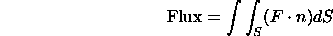# Magnetic flux with non-uniform magnetic field

• Northprairieman

#### Northprairieman

Hi there,

You know how magnetic flux through a loop of area A is the integral of B dot dA? If the area is perpendicular to the magnetic field, then we can write the dot product as B dA cos 0 = b dA. Also, if the magnetic field is uniform, the B can be brought out of the integral sign, and then the integral is just dA which is A. Thus, if the magnetic field is uniform and the area is perpendicular to the loop, the magnetic flux is just BA.

What I wanted to know is, what if the magnetic field is not uniform? What if it is decreasing by the square of the distance from the center of the loop? What I was trying to find is the magnetic flux at some distance r caused by a proton moving in a straight line.

The distance r is perpendicular to the proton's straight line path.

Hi Northprairieman,

one usually talks of flux of a vector field through a surface rather than through a loop. For a solenoidal field (null divergence) this flux depends only on the edge of the surface so I guess your concept is well-defined for a magnetic field.

The general form of a flux integral for a vector field F isoccasionally $$\textbf{n}dS$$ is written as $$d\textbf{S}$$; here n is the normal vector to the surface, which in general depends on the position on the surface.

To calculate this integral you have a couple of options:
- parametrize the surface and calculate the resulting 2-dim integral
- if the field is solenoidal, you express the field as curl of the vector potential and use Stokes theorem to transform the surface integral into a line integral on the edge of the surface

I understand that your loop is a circle centered on the moving charge, right?
Did you already calculate the field B or its vector potential?
Let me know if this is clear so far

Yes, thanks, that helps a lot. Yes, the loop is a circle centered on the moving charge. Am I right in assuming that the magnetic field decreases with the square of the distance from the moving charge?

I realized all I was looking for was the Biot-Savart law for a single particle instead of a current in a wire!

Hi,

neglecting non-stationary effects (retarded potentials etc), the magnetic field can be calculated with Lorentz transformations from the electrostatic field. E.g. see http://en.wikipedia.org/wiki/Classical_electromagnetism_and_special_relativity" [Broken]
At low velocity you get
B=v x E' /c^2
where E' is the electrostatic field of the proton

So yes the field falls with r^2 but it is not constant over a sphere of radius r because it depends on the angle between proton velocity and position vector.

In your case, flux could depend on the orientation of the circle (centered on the proton) wrt proton velocity.

Be careful if you were to choose an integration surface through the charge you will end up with a singularity.

The integral over an hemisphere is (very) easy to solve.

Let me know if you need details

Last edited by a moderator:
I realized all I was looking for was the Biot-Savart law for a single particle instead of a current in a wire!

Yup that works, same result (and same approximation)